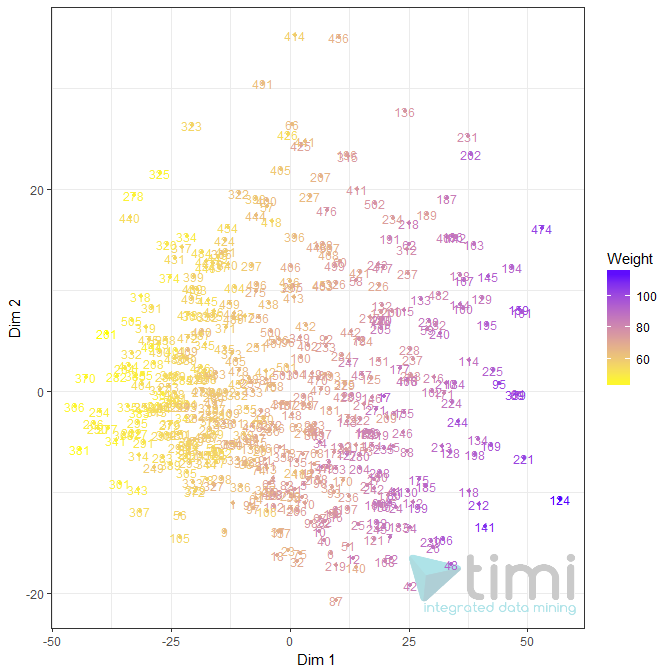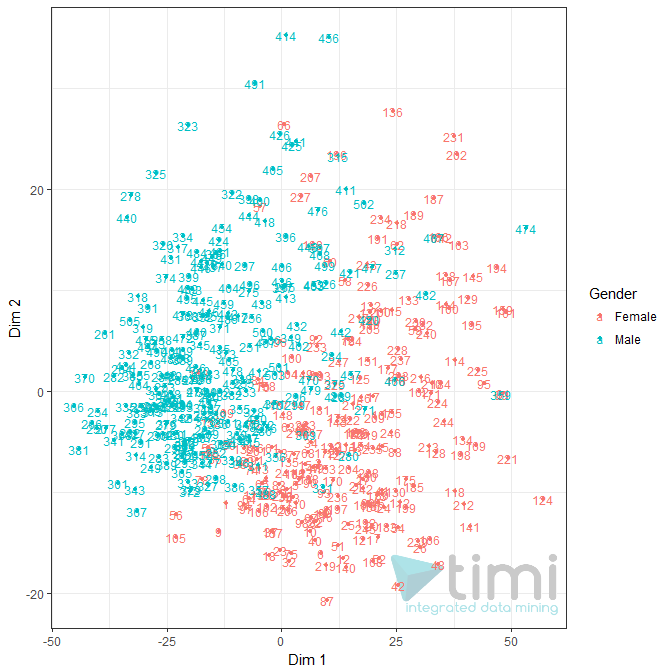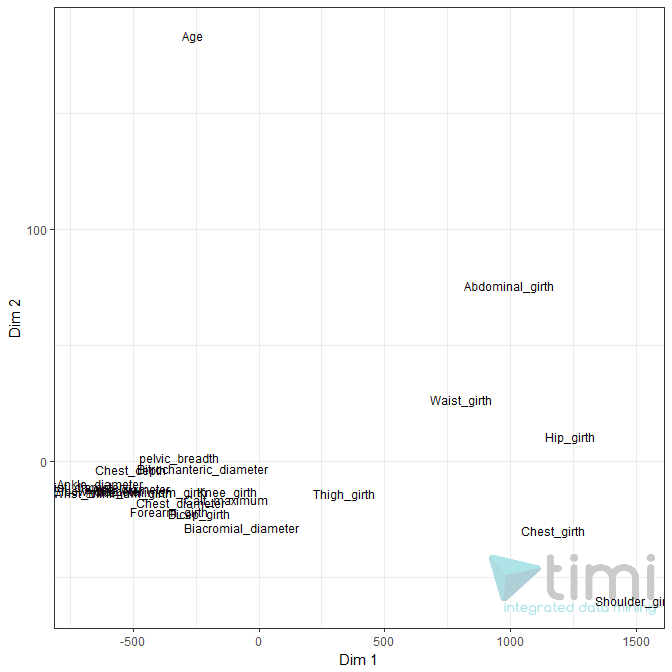﻿ 5. Detailed description of the Actions > 5.10. R Visualization > 5.10.9. Multi Dimensional Scaling (R action)

# 5.10.9. Multi Dimensional Scaling (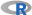action)

Icon: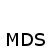Function: R_MultiDimensionalScaling

Property window: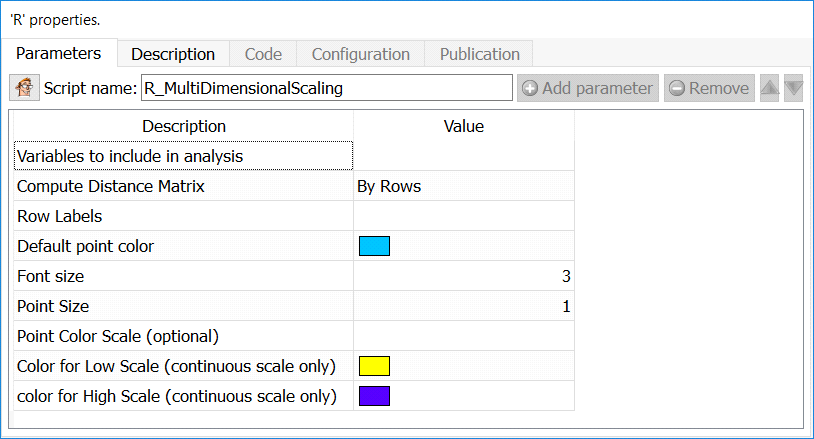Short description:

MDS is a visual data reduction method used to better understand the proximity between records, or between variables.

Long Description:

MDS is an algorithm based on similarity (Euclidean distance). The algorithm seeks to represents in 2-3 dimensions the best approximation of distance between points.

The implementation includes two modes: by row (similarity between observations) or by column (similarity between variables).

As this starts by generating a distance matrix, the algorithm quickly fills the memory when working with large database. It is, however, a good way to better understand correlation between columns (a bit like a PCA).

Variables to include in analysis: select the variables you wish to compute the distance on.

Compute Distance Matrix: Select whether you wish to study the distance between rows (observations) or columns (variables).

Row Labels: select the variable with the row lables (ID, etc.).

Default point color: select the color of the point on the chart (overruled if you used a scale variable).

Font Size: set the size of text displayed for point labels.

Point Size: set the size of points on the chart.

Point Color Scale: select a variable (nominal or continuous) to color the point and add additional information on the chart. Note that if the scale is NUMERICAL it MUST be set to float or key in Anatella, as R will consider a text vector of numbers as text (hence will treat it as categorical)

Color for Low Scale: if the color variable is a scale (ordinal or continuous), this will represent low values.

Color for High Scale: if the color variable is a scale (ordinal or continuous), this will represent High values.